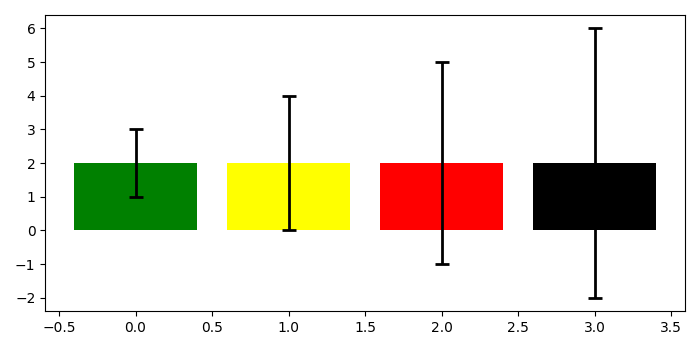# Setting different error bar colors in barplot in Matplotlib

To set different error bar colors in barplot in matplotlib, we can take the following steps −

• Create a figure and add a set of subplots using subplots() method.

• Make a barplot with data range 4, heights 2. yerr means vertical errorbars to the bar tips. The values are sizes relative to the data. Dictionary of kwargs to be passed to the errorbar method. Values of ecolor or capsize defined here take precedence over the independent kwargs.

• To display the figure, use show() method.

## Example

import matplotlib.pyplot as plt
plt.rcParams["figure.figsize"] = [7.00, 3.50]
plt.rcParams["figure.autolayout"] = True
fig, ax = plt.subplots()
ax.bar(range(4),  * 4, yerr=range(1, 5),
color=['green', 'yellow', 'red', 'black', 'orange'],
error_kw=dict(ecolor='black', lw=2, capsize=5, capthick=2))
plt.show()

## Output